Courses

Organometallic Compounds

20 Questions MCQ Test GATE Chemistry Mock Test Series | Organometallic Compounds

Description
Attempt Organometallic Compounds | 20 questions in 60 minutes | Mock test for Chemistry preparation | Free important questions MCQ to study GATE Chemistry Mock Test Series for Chemistry Exam | Download free PDF with solutions
QUESTION: 1

The complex (X) react with nucleophilies (R-) to give stable, neutral metal alkyl complex (Y). The product (Y) is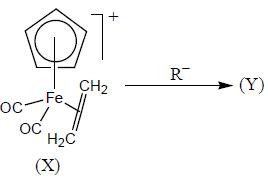Solution: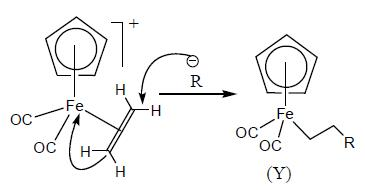QUESTION: 2

The 1HNMR spectrum of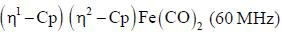at -800C and at 300C are respectively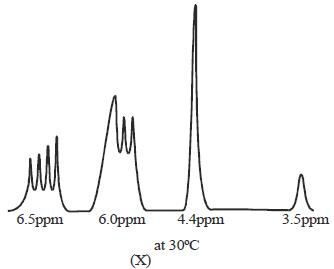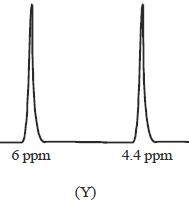Solution:

At high temperature(300C) due to fast 1,2-meta shift,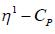mode looks like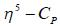mode. Hence, two signal. At low temperature, this rate is very slow, hence four signal corresponding to 4-types of hydrogen atom. Hence, (X and Y).

QUESTION: 3

The major product in the above reaction is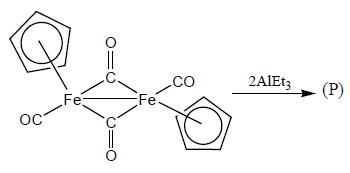Solution:

Bridging carbonyl is more basic than terminal hence Lewis acid attack at bridging carbonyl. Hence, correct option is (a)

QUESTION: 4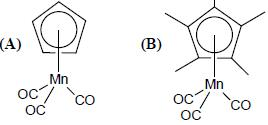The v c-o stretching frequency

Solution:

In B due to presence of methyl group, it will donate more electron density to metal. Hence, more back donation therefore, less value of v c-o.

QUESTION: 5

The compound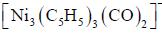has a single 'CO' stretching absorption at 1761cm-1. The IR data indicate that all C5H5 ligands are pentane to and probably in identical environment. On the basis of these data which of the following statement is true

Solution:

For all Cp to similar environment all the CO should be in μ3- mode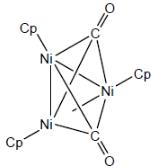QUESTION: 6

Bond lengths and angles have been determined by gas phase electron diffraction for two similar complexes, Fe(CO)4(C2H4)(X) and fe(CO)4(C2F4), (Y)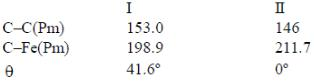Which of the following statement is true

Solution:

As C2F4 is strong -acceptor than C2H4. Hence, there will be more backbonding in (Y). Hence, C–C bond will be larger and M–C bond will be shorter in Y.

QUESTION: 7

The solved state magic angle spinning 13C NMR spectrum of Fe(CO)5 shows a single absorption at –26ºC but two singnals at –118ºC with relative intensity

Solution:

At –118ºC movement of axial ligand to equatorial is slow. Hence, axial and equatorial ligands give different signal. Hence, intensity ratio will be 2 : 3

*Answer can only contain numeric values
QUESTION: 8

Total number of isolobal fragments are_______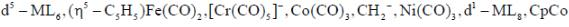Solution: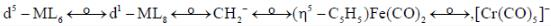*Answer can only contain numeric values
QUESTION: 9

Considering the quadnipolar nature of M -M bond in [Re2CI8]2-, M -M bond order in [Re2Cl4(PMe2Ph)4]is ___________

Solution:

[Re2Cl4(PMe2Ph)4]+
Let the oxidation states of two Re atoms are x and y
x + y - 4 = + 1
x + y = +5
x = +2, y = +3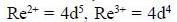Therefore, total number of d-electrons on Re2+ and Re3* ions = 5 + 4 = 9 Electronic configuration in this cluster ion is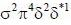Bond order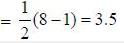*Answer can only contain numeric values
QUESTION: 10

Following the 18e- rule as a guide, determine x in the following complex.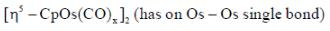Solution: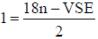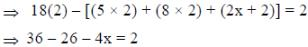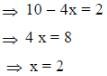QUESTION: 11

Which of the following statement is not true

Solution:

This reactions reductive elimination takes place first then followed by oxidative addition.

QUESTION: 12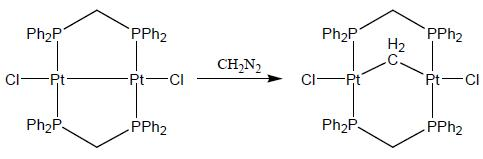The above reaction is an example of

Solution:

In above reaction CH2 get inserted  between two metal. Hence, reaction is an example of insertion reaction.

QUESTION: 13

Structures of Fe5(CO)15C and Os5(CO)16 respectively are

Solution: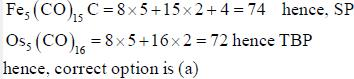QUESTION: 14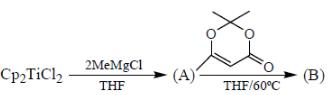Correct set representing A and B respectively are

Solution: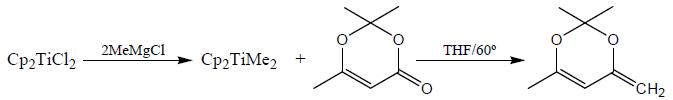QUESTION: 15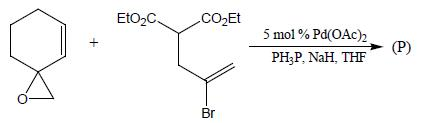Solution: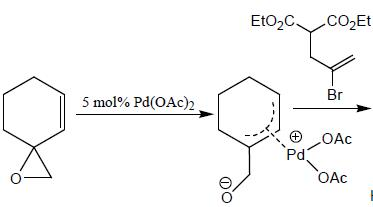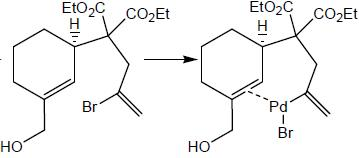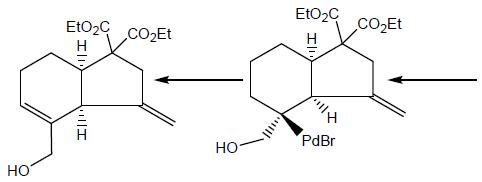QUESTION: 16

Consider the following reaction,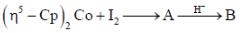Consider the correct statement regarding A and B
(1) complex A is diamagnetic in nature
(2) complex B contain one ligand in η4-mode
(3) A has smaller M-C bond length than(4) A has larger M-C bond length than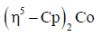Solution: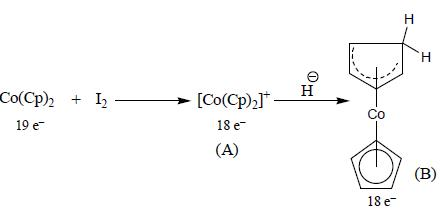Complex A obey 18 electron, in Co(Cp)there is an electron in antibonding orbital which leads to increase in bond length. Hence, correct option is (2) and (3).

QUESTION: 17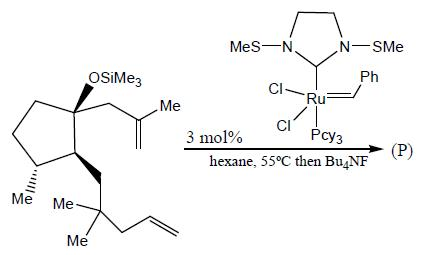Solution:

Grubbs catalyst used for ring closing metathesis of olefins.

*Answer can only contain numeric values
QUESTION: 18

The number of valence electrons provided by Co(CO)4 fragment towards cluster bonding is ___________

Solution:

Each transition metal has 12 electrons associated with it that are reserved for non-framework bonding. Electron more than 12 electrons are involved in cluster bonding.
Number of valence electron in Co(CO)4  =  17
Hence, number of electrons towards cluster bonding = 17–12 = 5

*Answer can only contain numeric values
QUESTION: 19

In the reaction shown below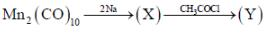Number of CO ligand in Y is_____

Solution: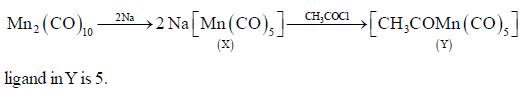*Answer can only contain numeric values
QUESTION: 20

The number of complexes having NO ligand in linear form are____.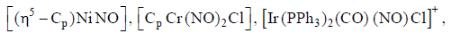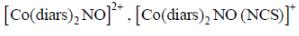Solution: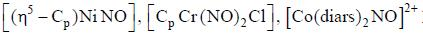have no ligand in linear form.Use Code STAYHOME200 and get INR 200 additional OFF Use Coupon Code

Track your progress, build streaks, highlight & save important lessons and more!

Similar ContentRelated tests